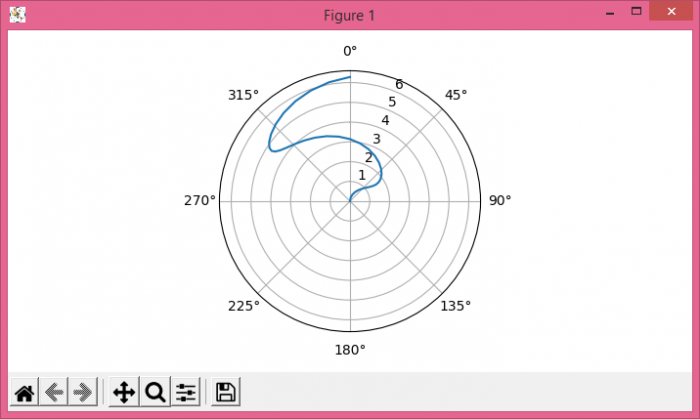# How to make the angles in a Matplotlib polar plot go clockwise with 0° at the top?

To make the angles in a matplotlib polar plot go clockwise with 00 at the top, we can take the followingsteps−

## Steps

• Add a subplot to the current figure ax.
• To set polar plot clockwise with top 0o, set the theta direction as −1 using set_theta_direction()method. And, use set_theta_offset() method to set the offset for the location of 0 in radians.
• Create theta, using numpy.
• Plot theta and sin(theta) on the current axis.
• To display the figure, use show() method.

## Example

import numpy as np
from matplotlib import pyplot as plt
plt.rcParams["figure.figsize"] = [7.00, 3.50]
plt.rcParams["figure.autolayout"] = True
ax = plt.subplot(1, 1, 1, projection='polar')
ax.set_theta_direction(-1)
ax.set_theta_offset(np.pi / 2.0)
theta = np.linspace(0, 2 * np.pi, 37)
ax.plot(np.sin(theta), theta)
plt.show()

## OutputUpdated on: 06-May-2021

3K+ Views